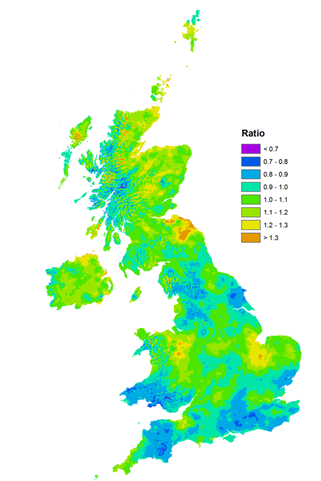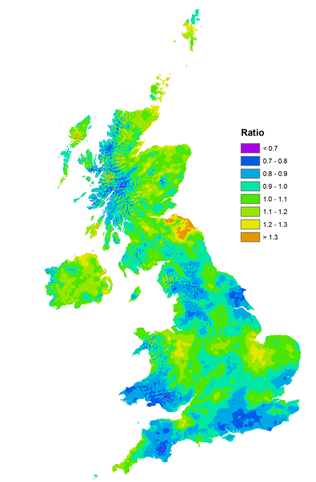# Design Rainfall Depth

The design rainfall depth is estimated as:

P = DDF \times ARF \times SCF

Where $DDF$ is the estimate of rainfall depth for a given duration and required frequency. The $ARF$ and $SCF$ are the areal reduction and seasonal correction factors that reflect the initial DDF model estimate is both a point estimate rather than a catchment estimate and is based on an analysis of annual maximum frequency rather than seasonal maximum frequency.

# Recommended DDF Rainfall Model

The FEH13 DDF model is the recommended rainfall model for use with ReFH2 although the software can be used with the legacy FEH99 DDF model. The FEH13 rainfall model is reported in detail by Stewart et al (2013) and the FEH99 DDF by Faulkner (1999).

This recommendation is based on the overall performance of the design packages, the differences in the spatial patterns in rainfall depths between the two models and the dependency of these on return period. The differences between the two rainfall models are illustrated in Figure 6. These results are the ratios of the FEH13 to the FEH99 rainfall depths for a 6 hour storm for the (1:100) and (1:200) return period.

There is a lot of detail within the spatial differences between the two models shown in these figures. However, the general patterns are that the FEH13 estimates tend to be lower (and by a greater amount) within areas of higher annual rainfall. In the drier areas of the UK the patterns are more mixed with greater rainfall depths observed in some areas, no change in others and lower depths in others. There is significant local detail in these patterns, for example some parts of the Scottish Highlands show significant increases in depths whereas the general pattern is one of general reductions in rainfall. Although not presented, the spatial patterns do not show a significant dependency on event duration. The consequence of these differences is that an adjustment term is required to ensure a correspondence between the return period of the design rainfall event and corresponding flow event when the FEH99 model is used.1:100 Year, 6 hour storm 1:200 Year, 6 hour storm

Figure 6. Ratio of the FEH13 to FEH99 rainfall depths for the 1:100 and 1:200 6 hour storm

# Areal Reduction Factors

The estimates of design rainfall calculated using the DDF model are point values as the model is based on data from individual gauges. To allow the estimation of catchment average design rainfall, the concept of the areal reduction factor ($ARF$) has been adopted from the existing FSR/FEH method. The FSR (NERC, 1975) originally defined and analysed the $ARF$ as “the ratio of rainfall depth over an $AREA$ to the rainfall depth of the same duration and return period at a representative point in the $AREA$”. The $FSR$ values of $ARF$ were adopted in the FEH.

The values used and those used in ReFH are those expressed mathematically by Keers and Wescott (1977) as:

ARF - 1-bD^{-a}

where $D$ is the duration of the design rainfall and $a$ and $b$ are the parameters presented on Table 3.

Table 3. Areal reduction factor parameters (Keers and Wescott, 1977)

AREA A (km²) A b
A ≤ 20 0.40 – 0.0208 ln[4.6–ln[A]] 0.0394 A$^{0.354}$
20 ≤ A < 100 0.40 – 0.00382 (4.6–ln[A])² 0.0394 A$^{0.354}$
100 ≤A < 500 0.40 – 0.00382 (4.6–ln[A])² 0.0627 A$^{0.254}$
500 ≤A < 1000 0.40 – 0.0208 ln[ln(A]–4.6) 0.0627 A$^{0.254}$
1000 ≤A 0.40 – 0.0208 ln(ln[A]–4.6) 0.1050 A$^{0.180}$

# Seasonal Correction Factors (SCF)

A seasonal correction factor was introduced within the ReFH method to support the use of summer and winter design inputs. The $SCF$ converts the $DDF$ estimate of design rainfall depth based on annual maximum rainfall into an estimate of seasonal design rainfall through simple multiplication. The $SCF$ is a correction factor depending on location, season, duration and selected return period. A detailed description of the development of the $SCF$ is given by Kjeldsen et al. (2006) with a shorter summary provided in the FEH Supplementary Report No1 (Kjeldsen, 2007).

The SCF was derived by fitting a GEV distribution to series of annual and seasonal maximum rainfall obtained from 523 daily raingauges and 172 subdaily recording raingauges located throughout the UK.

The $SCF$ for a given duration, $D$, are estimated using the following functional relationships:

SCF_D=\left\{\begin{matrix} \alpha SAAR + \beta & summer\\ (1-e^{[-\Phi SAAR]})^{\Psi} & winter \end{matrix}\right.

Where $SAAR$ is the catchment average value of the Standard period Average Annual Rainfall for the Meteorological Office 1961-1990 standard period. Please note the formulation is incorrect in the FEH Supplementary Report No1 (Kjeldsen, 2007).

Summer is defined as May to October and Winter as November to April.

For the summer relationship, a constraint was included in the parameter estimation that for $SAAR$ = 500 mm the seasonal correction factor equals one, i.e. 1 = $\alpha$ 500 mm + $\beta$. The parameters for duration of 1, 2, 6 and 24 hours are presented on Table 4. Values for other durations in the range [1,24] hrs are estimated using linear interpolation between adjacent values.

Table 4. Seasonal correction factor parameters

Summer Summer Winter Winter
Duration $\alpha$ $\beta$ $\Phi$ $\Psi$
≤1 hour –8.03x10$^{-5}$ 1.04 0.0004 0.4000
2 hours –6.87x10$^{-5}$ 1.03 0.0006 0.4454
6 hours –4.93x10$^{-5}$ 1.02 0.0009 0.4672
≥ 24 hours –10.26x10$^{-5}$ 1.05 0.0011 0.5333

Other than at the extremes of $SAAR$, where the tendency may be for the majority of annual maxima to lie within a particular season, annual maxima can occur in the other season. The seasonal rainfall will therefore tend be lower than the annual counterpart. The SCF is an integral part of the ReFH method. The application of the SCF in other models will depend on the context within which the rainfall data is used.